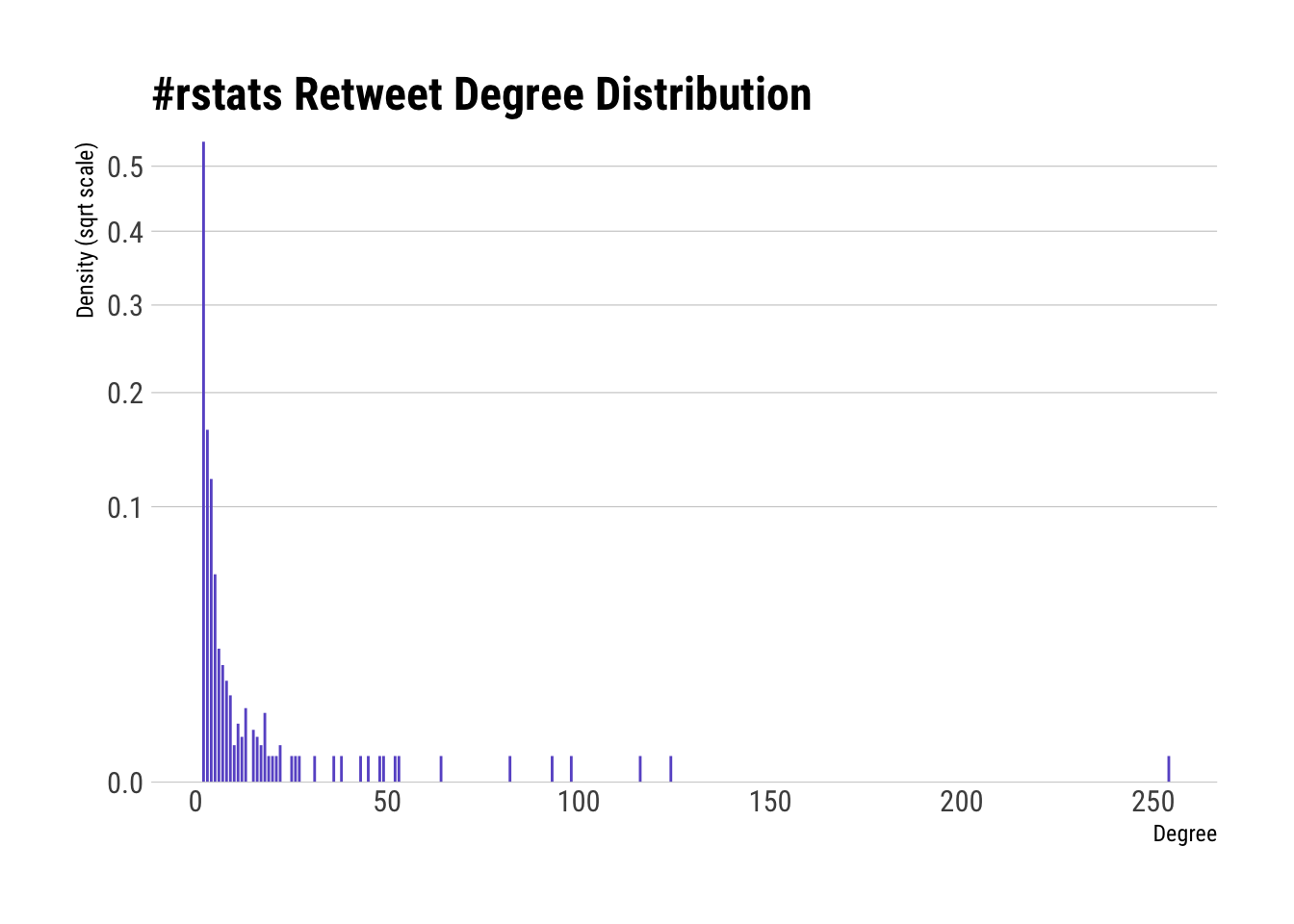# Recipe 6 Creating a Graph of Retweet Relationships

## 6.1 Problem

You want to construct and analyze a graph data structure of retweet relationships for a set of query results.

## 6.2 Solution

Query for the topic, extract the retweet origins, and then use `igraph` to construct a graph to analyze.

## 6.3 Discussion

Recipes 4 and 5 introduced and expanded on searching Twitter plus looking for retweets. The `igraph` package can be used to capture and analyze details of relationships across retweets. We’ll focus on just examining the Twitter user pair relationships.

Let’s get a larger sample this time — 1,500 tweets in `#rstats`. We can use the technique from the previous recipe and:

• find the retweets (using the API-provided data)
• expand out all the mentioned screen names
• create an `igraph` graph object
• look at some summary statistics for the graph
``````library(rtweet)
library(igraph)
library(hrbrthemes)
library(tidyverse)``````
``````rstats <- search_tweets("#rstats", n=1500)

filter(rstats, retweet_count > 0) %>%
select(screen_name, mentions_screen_name) %>%
unnest(mentions_screen_name) %>%
filter(!is.na(mentions_screen_name)) %>%
graph_from_data_frame() -> rt_g``````

You can reference the `igraph` `print()` and `summary()` functions for more information on the output of `summary()` but output from the following line shows that the graph is `D`irected with `N`amed vertices and it has 1,106 vertices and 1,945 edges.

``summary(rt_g)``
``````## IGRAPH b4b8447 DN-- 1106 1945 --
## + attr: name (v/c)``````

We’ll produce more visualizations in the next recipe, but the degree of graph vertices is one of the most fundamental properties of a graph and it’s much nicer to see the degree distribution than stare at a wall of numbers:

``````ggplot(data_frame(y=degree_distribution(rt_g), x=1:length(y))) +
geom_segment(aes(x, y, xend=x, yend=0), color="slateblue") +
scale_y_continuous(expand=c(0,0), trans="sqrt") +
labs(x="Degree", y="Density (sqrt scale)", title="#rstats Retweet Degree Distribution") +
theme_ipsum_rc(grid="Y", axis="x")``````